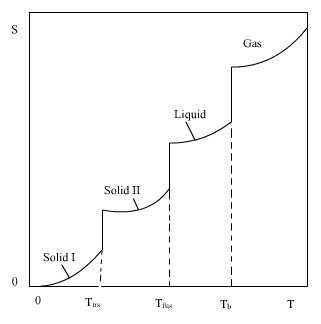## The Third Law of Thermodynamics and Absolute Energy

#### Learning Objective

• Recall the third law of thermodynamics.

#### Key Points

• At zero temperature the system must be in a state with the minimum thermal energy.
• Mathematically, the absolute entropy of any system at zero temperature is the natural log of the number of ground states times Boltzmann’s constant kB.
• For the entropy at absolute zero to be zero, the magnetic moments of a perfectly ordered crystal must themselves be perfectly ordered.

#### Terms

• third law of thermodynamicsa law which states that the entropy of a perfect crystal at absolute zero is exactly equal to zero
• paramagneticattracted to the poles of a magnet
• ferromagneticthe basic mechanism by which certain materials form permanent magnets, or are attracted to magnets

## The Basic Law

The third law of thermodynamics states that the entropy of a system approaches a constant value as the temperature approaches zero. The entropy of a system at absolute zero is typically zero, and in all cases is determined only by the number of different ground states it has. Specifically, the entropy of a pure crystalline substance at absolute zero temperature is zero.

At zero temperature the system must be in a state with the minimum thermal energy. This statement holds true if the perfect crystal has only one state with minimum energy. Entropy is related to the number of possible microstates according to [latex]S = k_Bln(\Omega)[/latex], where S is the entropy of the system, kB is Boltzmann’s constant, and Ω is the number of microstates (e.g. possible configurations of atoms).

At absolute zero there is only 1 microstate possible (Ω=1) and ln(1) = 0. A more general form of the third law applies to systems such as glasses that may have more than one minimum energy state: the entropy of a system approaches a constant value as the temperature approaches zero. The constant value (not necessarily zero) is called the residual entropy of the system. Physically, the law implies that it is impossible for any procedure to bring a system to the absolute zero of temperature in a finite number of steps.Entropy as a Function of TemperatureThe entropy (S) of a substance (compound or element) as a function of temperature (T).

## Absolute Entropy

The entropy determined relative to this point (absolute zero) is the absolute entropy. Mathematically, the absolute entropy of any system at zero temperature is the natural log of the number of ground states times Boltzmann’s constant kB. The entropy of a perfect crystal lattice is zero, provided that its ground state is unique (only one), because ln(1) = 0.

An example of a system which does not have a unique ground state is one containing half-integer spins, for which there are two degenerate ground states. For such systems, the entropy at zero temperature is at least ln(2)kB, which is negligible on a macroscopic scale.

In addition, glasses and solid solutions retain large entropy at absolute zero, because they are large collections of nearly degenerate states, in which they become trapped out of equilibrium. For the entropy at absolute zero to be zero, the magnetic moments of a perfectly ordered crystal must themselves be perfectly ordered. Only ferromagnetic, antiferromagnetic, and diamagnetic materials can satisfy this condition. Materials that remain paramagnetic at absolute zero, by contrast, may have many nearly-degenerate ground states, as in a spin glass, or may retain dynamic disorder, as is the case in a spin liquid.